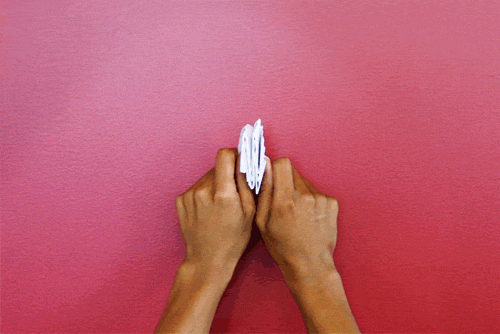# Not Just Geometry! It's Physics!Consider the animation above, where the rectangular paper is folded and then unfolded repeatedly. They are both done by controlling the opposite vertices of the rectangular paper in a single move.

Assume that the repeating polygons have the same areas and are symmetric. Also assume that horizontal parallel lines are perpendicular to the paper's edges. Which of the following best illustrate the interior creased polygons (including those bounded by the paper's edges) in the animation after unfolding the paper?

Clarification:

• Mix of quadrilaterals refer to class of 4-sided polygons not specified in the options.
• A trapezoid is a quadrilateral with a pair of parallel sides. It is also known as a trapezium in UK.
×